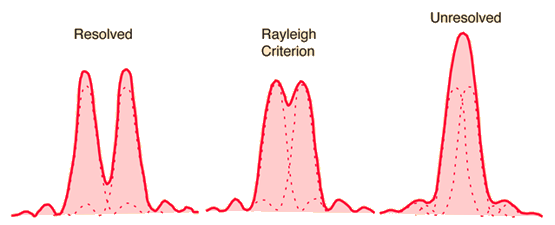## IB Physics Glossary

 Search full text

Browse the glossary using this index

Special | A | B | C | D | E | F | G | H | I | J | K | L | M | N | O | P | Q | R | S | T | U | V | W | X | Y | Z | ALL

### R

occurs when a nucleus emits an $$\alpha$$-particle/ $$\beta$$-particle/ $$\gamma$$-particle /ionizing radiations.
The process is random and spontaneous since it is unknown when a nucleus will decay.
The activity is proportional to the number of undecayed nuclei.
The nucleus becomes more (energetically) stable.
There is a constant probability of decay.
The activity/number of unstable nuclei in sample reduces by half over every half-life.
It is not affected by temperature/environmental conditions.
 Keyword(s): 7.2

is written as an exponential decay function
$$N=N_0 e^{-\lambda t}$$
N: #nuclei at time t
N0: initial number of nuclei
$$\lambda$$: the decay constant - probability of a decay per unit time
t: time

Note
$$A = - \frac{dN}{dt}= \lambda N$$
or
$$A = A_0 e^{-\lambda t}$$
where $$A_0 = \lambda N_0$$
A: activity at time t
A0: initial activity of sample
$$\lambda$$: the decay constant - probability of a decay per unit time
t: time

 Keyword(s): 13.2

#### Random error

is a fluctuating error often present in experiments. It is linked to precision: imprecise data => "high" random error; precise data => "low" random error. Repeated measurements do reduce random errors. Sources of random errors can include varying reading/human error and other randomly flucuating factors which cannot be controlled during experiments.
 Keyword(s): 1.2

#### Rayleigh Criterion

for images of two wave sources to be just resolved the maximum of one diffraction pattern is coincident with the first minimum of the other. (d)The minimum anglular separation in the diffraction pattern, $$\theta_{min}$$ for two objects to be resolved is given by

$$\theta_{min} = \frac{\lambda}{b}$$, for single slits of width b;

$$\theta_{min} = 1.22 \frac{\lambda}{b}$$, for circular apertures of diameter b.

If two objects at a distance r from the pupil/telescope, separated by distance s, are just resolved then, since $$s=r \theta$$, we can write

$$1.22\frac{\lambda}{b} = \frac{s}{r}$$

which can be used to solve typical problems on resolution.

 Keyword(s): 9

#### Resisitvity

can be found from the resisitivity equation

$$R = \frac{\rho L}{A}$$,
where R is the resitance, $$\rho$$ is the resistivity, L is the length and A is the cross-sectional area, $$A=\pi r^2=\pi \frac{d^2}{4}$$.

<< Simulation >>

 Keyword(s): 5.1

#### Resistance

is the ratio of voltage to current, $$R =\frac{V}{I}$$. (d)
 Keyword(s): 5.2

#### Resistance combinations

occur when resistances or components are placed in series and/or in parallel combinations. The main differences between the two types of combinations are

 Series Parallel DiagramCurrent same current through each resistor current splits to flow through each resistor; total current through the whole circuit is the sum of the currents through the separate resistors Potential difference potential difference is split between the resistors - refer to potential divider rule. potential difference across each resistor is the same Resistance resistance increases, current decreases, when resistors are combined in series resistance decreases, current increases, when resistors are combined in parallel Other properties current has one path to flow through; if one resistor breaks, current stops flowing current has more than one path to flow through; if one resistor breaks, current can still flow through other resistors Formulae $$R =R_1 + R_2 + \ldots + R_n$$ $$\frac{1}{R}= \frac{1}{R_1} + \frac{1}{R_2} + \ldots +\frac{1}{R_n}$$For pairs this reduces to$$R = \frac{R_1 \times R_2}{R_1 + R_2}$$ie. product over sumFor identical resistances in parallel, $$R_1=R_2$$, $$R = \frac{R_1}{2}$$ie. resistance value halves

 Keyword(s): 5.2

#### Resonance

occurs when an object is forced to vibrate at its natural frequency with a very large increase in amplitude. (d)

Resonance is the opposite to damping.If external driving frequency, f, equals to the natural frequency, f0, then
f = f0 or f/f0 = 1, and resonance occurs.Notice the resonance amplitude decreases with damping, and the natural frequency decreases.
 Keyword(s): 4.3

#### rms voltage or current

equals $$\frac{1}{\sqrt 2}$$ times the peak voltage or current. (s)
 Keyword(s): 12.2